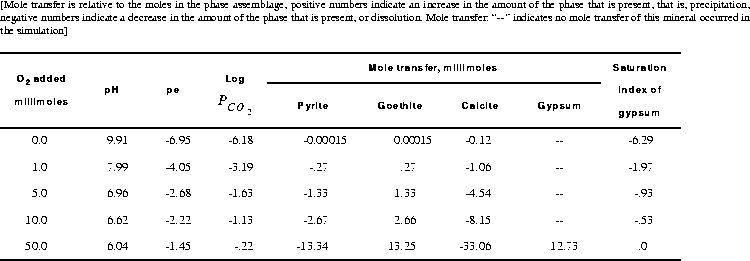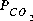[Next] [Previous] [Up] [Top]

EXAMPLES

## Example 5.-- Irreversible Reactions

This example demonstrates the irreversible reaction capabilities of PHREEQC in modeling the oxidation of pyrite. Oxygen is added irreversibly to pure water in five varying amounts (0.0, 1.0, 5.0, 10.0, and 50.0 mmol), while pyrite, calcite, and goethite are allowed to dissolve to equilibrium. In addition, gypsum is allowed to precipitate if it becomes supersaturated

### Table 11. Input data set for example 5

```TITLE Example 5.--Add oxygen, equilibrate with pyrite, calcite, and goethite.
SOLUTION 1  PURE WATER
pH      7.0
temp    25.0
EQUILIBRIUM_PHASES 1
Pyrite          0.0
Goethite        0.0
Calcite         0.0
Gypsum          0.0     0.0
REACTION 1
O2      1.0
0.0     0.001   0.005   0.01    0.05
SELECTED_OUTPUT
-file   ex5.pun
-si     CO2(g)  Gypsum
-equilibrium_phases  pyrite goethite calcite gypsum
END
```
Pure water is defined with SOLUTION input (table 11), and the pure-phase assemblage is defined with EQUILIBRIUM_PHASES input. Because gypsum has an initial amount of 0.0 mol, gypsum can only precipitate if it becomes supersaturated; it can not dissolve because no moles are present. The REACTION data block defines the irreversible reaction that is to be modeled. In this example, oxygen ("O2") will be added with a relative fraction of 1.0. The steps of the reaction are defined to be 0.0, 0.001, 0.005, 0.01, and 0.05 mol. The reactants can be defined by a chemical formula, as in this case (O2) or by a phase name that has been defined with PHASES input. Thus, the phase name "O2(g)" from the default database file, could have been used in place of "O2" to achieve the same result. The number of moles of the element oxygen (as O, not O2) added in each reaction step is equal to the stoichiometric coefficient of oxygen in O2 (2) times the relative fraction (1.0) times the number of moles in the reaction step. The relative fraction is useful in reactions that have multiple reactants because it defines the relative rates of reaction among the reactants. SELECTED_OUTPUT was used to write the partial pressure of carbon dioxide, the saturation index of gypsum, and the total amounts and mole transfers of pyrite, goethite, calcite, and gypsum to the file ex5.pun after each equilibrium calculation.

### Table 12. Selected results for example 5.The results for example 5 are summarized in table 12. When no oxygen is added to the system, a small amount of calcite dissolves and trace amounts of pyrite and goethite react; the pH is relatively high (9.91), the pe is low (-6.95), and logis low (-6.18). As oxygen is added, pyrite is oxidized and goethite, being relatively insoluble, precipitates. This generates sulfuric acid, decreases the pH, and causes calcite to dissolve. During these reactions, the pe and logincrease. At some point between 10 and 50 mmol of oxygen added, gypsum reaches saturation and begins to precipitate. When 50 mmol of oxygen have been added, a total of 12.73 mmol of gypsum has precipitated. After 1 or more millimoles of oxygen have been added, theis much greater than atmospheric (10-3.5 atm). If the system is assumed to be open to the atmosphere, carbon dioxide should be included as one of the equilibrium phases with a target partial pressure of atmospheric, which would allow the simulated release of carbon dioxide to the atmosphere

Table 11. Input data set for example 5
Table 12. Selected results for example 5.

User's Guide to PHREEQC - 07 MAY 96
[Next] [Previous] [Up] [Top]